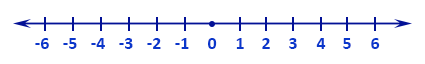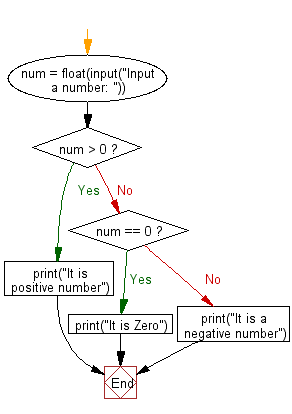﻿ Python: Check if a number is positive, negative or zero - w3resource# Python: Check if a number is positive, negative or zero

## Python Basic: Exercise-109 with Solution

Write a Python program to check if a number is positive, negative or zero.

Positive Numbers: Any number above zero is known as a positive number. Positive numbers are written without any sign or a '+' sign in front of them and they are counted up from zero, i.e. 1, + 2, 3, +4 etc.
Negative Numbers: Any number below zero is known as a negative number. Negative numbers are always written with a '−' sign in front of them and they are counted down from zero, i.e. -1, -2, -3, -4 etc.
Always look at the sign in front of a number to check if it is positive or negative. Zero, 0, is neither positive nor negative.

Pictorial Presentation:Sample Solution-1:

Python Code:

``````num = float(input("Input a number: "))
if num > 0:
print("It is positive number")
elif num == 0:
print("It is Zero")
else:
print("It is a negative number")
```
```

Sample Output:

```Input a number: 150
It is positive number
```

Flowchart:## Visualize Python code execution:

The following tool visualize what the computer is doing step-by-step as it executes the said program:

Sample Solution-2:

Python Code:

``````n = float(input('Input a number: '))
print('Number is Positive.' if n > 0 else 'It is Zero!' if n == 0 else 'Number is Negative.')
```
```

Sample Output:

```Input a number:  0
It is Zero!
```

## Visualize Python code execution:

The following tool visualize what the computer is doing step-by-step as it executes the said program:

Sample Solution-3:

Python Code:

``````n = float(input("Input a number: "))
if n >= 0:
if n == 0:
print("It is Zero!")
else:
print("Number is Positive number.")
else:
print("Number is Negative number.")
```
```

Sample Output:

```Input a number:  -150
Number is Negative number.
```

## Visualize Python code execution:

The following tool visualize what the computer is doing step-by-step as it executes the said program:

Python Code Editor:

Have another way to solve this solution? Contribute your code (and comments) through Disqus.

What is the difficulty level of this exercise?

Test your Python skills with w3resource's quiz

﻿

## Python: Tips of the Day

Try-catch-else construct:

```try:
foo()
except Exception:
print("Exception occured")
else:
print("Exception didnt occur")
finally:
print("Always gets here")
```